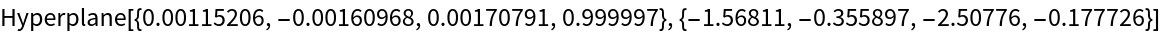Function Repository Resource:

PlaneOfBestFit

Get the hyperplane that best fits a set of points

Contributed by: Jason Biggs
 ResourceFunction["PlaneOfBestFit"][{p1,p2,…}] returns an InfinitePlane that minimizes the sum of squares of orthogonal distances to the 3D points pi.

Details

In two dimensions, ResourceFunction["PlaneOfBestFit"] returns an InfiniteLine.
In dimensions higher than three, ResourceFunction["PlaneOfBestFit"] returns a Hyperplane.

Examples

Basic Examples (3)

Generate a set of random points in space:

 In:=Out=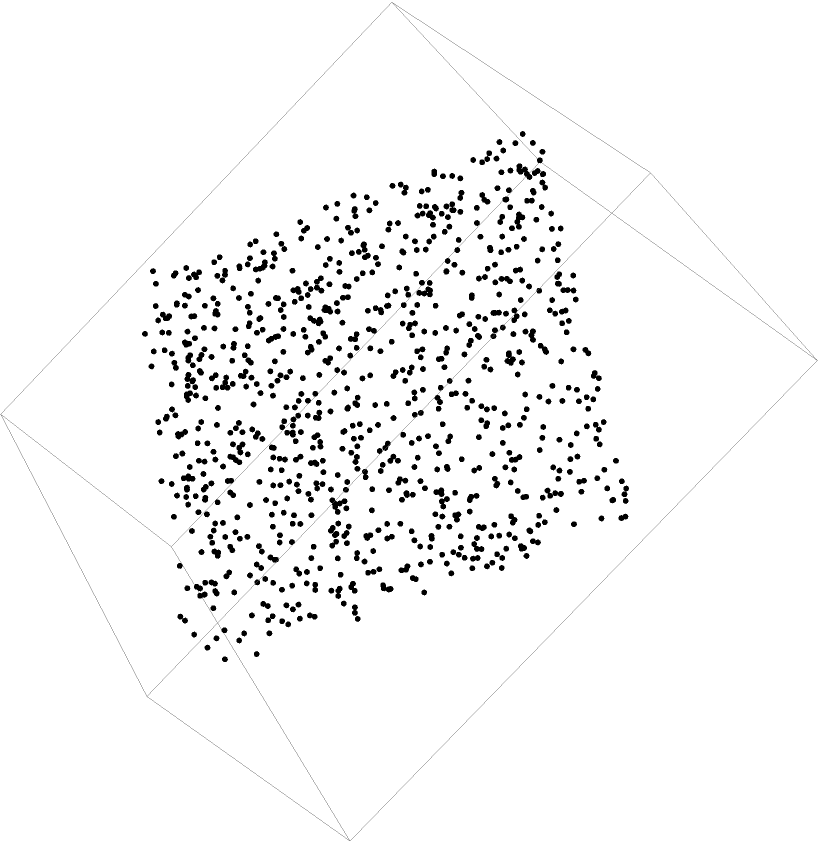Find the plane of best fit:

 In:=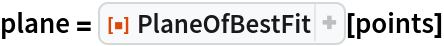Out=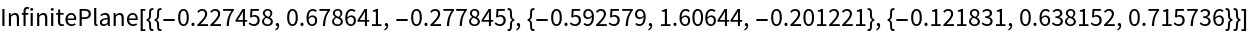Show the best­fit plane along with the points:

 In:=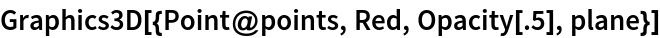Out=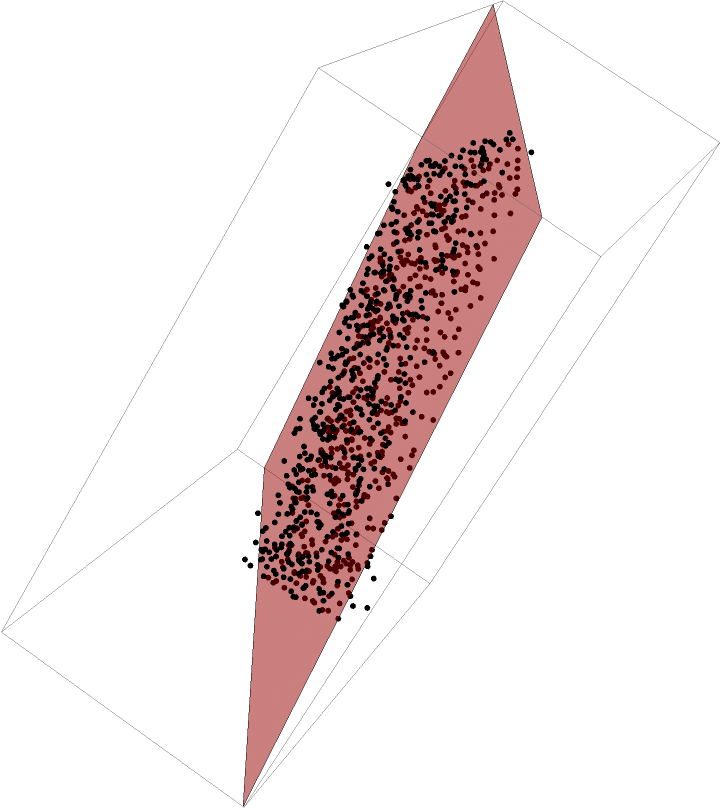Scope (2)

Create a best-fit line for points in 2D. First generate a set of random data:

 In:=Out=Find the line of best fit:

 In:=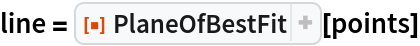Out=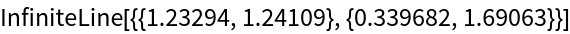Show the best­fit line along with the points:

 In:=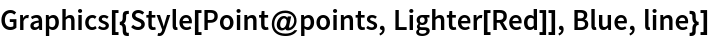Out=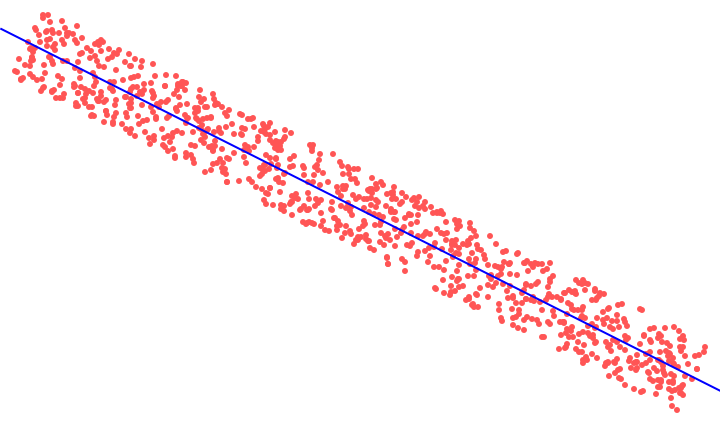Create points in 4D such that the spread along the last axis is much less than along the other three:

 In:=Create a best-fit 3D hyperplane to these points and obtain a normal vector close to {0,0,0,1}:

 In:=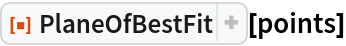Out=